Chapter 10 Geometric Optics

# 10.3 The Law of Refraction – Snell’s Law

### Summary

• Determine the index of refraction, given the speed of light in a medium.

It is easy to notice some odd things when looking into a fish tank. For example, you may see the same fish appearing to be in two different places. (See Figure 1.) This is because light coming from the fish to us changes direction when it leaves the tank, and in this case, it can travel two different paths to get to our eyes. The changing of a light ray’s direction (loosely called bending) when it passes through variations in matter is called refraction. Refraction is responsible for a tremendous range of optical phenomena, from the action of lenses to voice transmission through optical fibers.

### Refraction

The changing of a light ray’s direction (loosely called bending) when it passes through variations in matter is called refraction.

### Speed of Light

The speed of light not only affects refraction, it is one of the central concepts of Einstein’s theory of relativity. As the accuracy of the measurements of the speed of light were improved, was found not to depend on the velocity of the source or the observer. However, the speed of light does vary in a precise manner with the material it traverses. These facts have far-reaching implications, as we will see in Chapter on Special Relativity. It makes connections between space and time and alters our expectations that all observers measure the same time for the same event, for example. The speed of light is so important that its value in a vacuum is one of the most fundamental constants in nature as well as being one of the four fundamental SI units.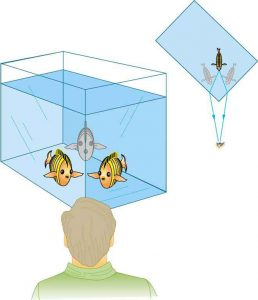Figure 1. Looking at the fish tank as shown, we can see the same fish in two different locations, because light changes directions when it passes from water to air. In this case, the light can reach the observer by two different paths, and so the fish seems to be in two different places. This bending of light is called refraction and is responsible for many optical phenomena.

Why does light change direction when passing from one material (medium) to another? It is because light changes speed when going from one material to another. So before we study the law of refraction, it is useful to discuss the speed of light and how it varies in different media.

# The Speed of Light

Early attempts to measure the speed of light, such as those made by Galileo, determined that light moved extremely fast, perhaps instantaneously. The first real evidence that light traveled at a finite speed came from the Danish astronomer Ole Roemer in the late 17th century. Roemer had noted that the average orbital period of one of Jupiter’s moons, as measured from Earth, varied depending on whether Earth was moving toward or away from Jupiter. He correctly concluded that the apparent change in period was due to the change in distance between Earth and Jupiter and the time it took light to travel this distance. From his 1676 data, a value of the speed of light was calculated to be 2.26 x 108 m/s (only 25% different from today’s accepted value). In more recent times, physicists have measured the speed of light in numerous ways and with increasing accuracy. One particularly direct method, used in 1887 by the American physicist Albert Michelson (1852–1931), is illustrated in Figure 2. Light reflected from a rotating set of mirrors was reflected from a stationary mirror 35 km away and returned to the rotating mirrors. The time for the light to travel can be determined by how fast the mirrors must rotate for the light to be returned to the observer’s eye.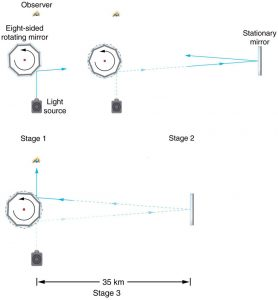Figure 2. A schematic of early apparatus used by Michelson and others to determine the speed of light. As the mirrors rotate, the reflected ray is only briefly directed at the stationary mirror. The returning ray will be reflected into the observer’s eye only if the next mirror has rotated into the correct position just as the ray returns. By measuring the correct rotation rate, the time for the round trip can be measured and the speed of light calculated. Michelson’s calculated value of the speed of light was only 0.04% different from the value used today.

The speed of light is now known to great precision. In fact, the speed of light in a vacuum,    , is so important that it is accepted as one of the basic physical quantities and has the fixed value

c = 2.9972458 x 108 m/s   ≈  3.00 x 108 m/s

where the approximate value of 3.00 x 108 m/s is used whenever three-digit accuracy is sufficient. The speed of light through matter is less than it is in a vacuum, because light interacts with atoms in a material. The speed of light depends strongly on the type of material, since its interaction with different atoms, crystal lattices, and other substructures varies. We define the index of refraction n  of a material to be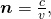where v is the observed speed of light in the material. Since the speed of light is always less than c in matter and equals only in a vacuum, the index of refraction is always greater than or equal to one.

### Value of the Speed of Light

c = 2.9972458 x 108  m/s  ≈ 3.00 x 108  m/s

### Index of Refraction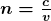That is,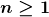. Table 1 gives the indices of refraction for some representative substances. The values are listed for a particular wavelength of light, because they vary slightly with wavelength. (This can have important effects, such as colours produced by a prism.) Note that for gases, n is close to 1.0. This seems reasonable, since atoms in gases are widely separated and light travels at c in the vacuum between atoms. It is common to take n=1 for gases unless great precision is needed. Although the speed of light in a medium varies considerably from its value in a vacuum, it is still a large speed.

Medium n
Gases at 0ºC, 1 atm
Air 1.000293
Carbon dioxide 1.00045
Hydrogen 1.000139
Oxygen 1.000271
Liquids at 20ºC
Benzene 1.501
Carbon disulfide 1.628
Carbon tetrachloride 1.461
Ethanol 1.361
Glycerine 1.473
Water, fresh 1.333
Solids at 20ºC
Diamond 2.419
Fluorite 1.434
Glass, crown 1.52
Glass, flint 1.66
Ice at 20ºC 1.309
Polystyrene 1.49
Plexiglas 1.51
Quartz, crystalline 1.544
Quartz, fused 1.458
Sodium chloride 1.544
Zircon 1.923
Table 1: Index of Refraction in Various Media

### Example 1: Speed of Light in Matter

Calculate the speed of light in zircon, a material used in jewelry to imitate diamond.

Strategy

The speed of light in a material, v, can be calculated from the index of refraction, n,  of the material using the equation n = c / v .

Solution

The equation for index of refraction states that n=c/v. Rearranging this to determine v gives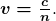The index of refraction for zircon is given as 1.923 in Table 1, and c is given in the equation for speed of light. Entering these values in the last expression gives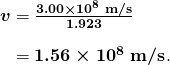Discussion

This speed is slightly larger than half the speed of light in a vacuum and is still high compared with speeds we normally experience. The only substance listed in Table 1 that has a greater index of refraction than zircon is diamond. We shall see later that the large index of refraction for zircon makes it sparkle more than glass, but less than diamond.

# Law of Refraction

Figure 3 shows how a ray of light changes direction when it passes from one medium to another. As before, the angles are measured relative to a perpendicular to the surface at the point where the light ray crosses it. (Some of the incident light will be reflected from the surface, but for now we will concentrate on the light that is transmitted.) The change in direction of the light ray depends on how the speed of light changes. The change in the speed of light is related to the indices of refraction of the media involved. In the situations shown in Figure 3, medium 2 has a greater index of refraction than medium 1. This means that the speed of light is less in medium 2 than in medium 1. Note that as shown in Figure 3(a), the direction of the ray moves closer to the perpendicular when it slows down. Conversely, as shown in Figure 3(b), the direction of the ray moves away from the perpendicular when it speeds up. The path is exactly reversible. In both cases, you can imagine what happens by thinking about pushing a lawn mower from a footpath onto grass, and vice versa. Going from the footpath to grass, the front wheels are slowed and pulled to the side as shown. This is the same change in direction as for light when it goes from a fast medium to a slow one. When going from the grass to the footpath, the front wheels can move faster and the mower changes direction as shown. This, too, is the same change in direction as for light going from slow to fast.Figure 3. The change in direction of a light ray depends on how the speed of light changes when it crosses from one medium to another. The speed of light is greater in medium 1 than in medium 2 in the situations shown here. (a) A ray of light moves closer to the perpendicular when it slows down. This is analogous to what happens when a lawn mower goes from a footpath to grass. (b) A ray of light moves away from the perpendicular when it speeds up. This is analogous to what happens when a lawn mower goes from grass to footpath. The paths are exactly reversible.

The amount that a light ray changes its direction depends both on the incident angle and the amount that the speed changes. For a ray at a given incident angle, a large change in speed causes a large change in direction, and thus a large change in angle. The exact mathematical relationship is the law of refraction, or “Snell’s Law,” which is stated in equation form as

n1 sin θ1 = n2 sin θ2

Here n1 and n2 are the indices of refraction for medium 1 and 2, and θ1 and θ2 are the angles between the rays and the perpendicular in medium 1 and 2, as shown in Figure 3. The incoming ray is called the incident ray and the outgoing ray the refracted ray, and the associated angles the incident angle and the refracted angle. The law of refraction is also called Snell’s law after the Dutch mathematician Willebrord Snell (1591–1626), who discovered it in 1621. Snell’s experiments showed that the law of refraction was obeyed and that a characteristic index of refraction could be assigned to a given medium. Snell was not aware that the speed of light varied in different media, but through experiments he was able to determine indices of refraction from the way light rays changed direction.

### The Law of Refraction

n1 sin θ1 = n2 sin θ2

### Take-Home Experiment: A Broken Pencil

A classic observation of refraction occurs when a pencil is placed in a glass half filled with water. Do this and observe the shape of the pencil when you look at the pencil sideways, that is, through air, glass, water. Explain your observations. Draw ray diagrams for the situation.

### Example 2: Determine the Index of Refraction from Refraction Data

Find the index of refraction for medium 2 in Figure 3(a), assuming medium 1 is air and given the incident angle is 30.0 degrees and the angle of refraction is 22.0 degrees.

Strategy

The index of refraction for air is taken to be 1 in most cases (and up to four significant figures, it is 1.000). Thus  n1 = 1.00 here. From the given information, θ1 = 30.0o and the second angle = 22.0  degrees. With this information, the only unknown in Snell’s law is n2 so that it can be used to find this unknown.

Solution

Snell’s law is

n1 sin θ1 = n2 sin θ2

Rearranging to isolate n2  gives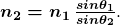Entering known values,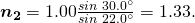Discussion

This is the index of refraction for water, and Snell could have determined it by measuring the angles and performing this calculation. He would then have found 1.33 to be the appropriate index of refraction for water in all other situations, such as when a ray passes from water to glass. Today we can verify that the index of refraction is related to the speed of light in a medium by measuring that speed directly.

### Example 3: A Larger Change in Direction

Suppose that in a situation like that in Example 2, light goes from air to diamond and that the incident angle is 30.0o. Calculate the angle of refraction θ2 in the diamond.

Strategy

Again the index of refraction for air is taken to be n1 = 1.00, and we are given θ1 = 30.0 o. We can look up the index of refraction for diamond in Table 1, finding n2 = 2.419. The only unknown in Snell’s law is θ2, which we wish to determine.

Solution

Solving Snell’s law for sin θ2 yields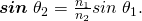Entering known values,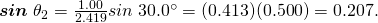The angle is thus

θ2 = sin-1 (0.207) = 11.9 o

Discussion

For the same 30.0o angle of incidence, the angle of refraction in diamond is significantly smaller than in water 11.9o rather than 22.0o—see the preceding example). This means there is a larger change in direction in diamond. The cause of a large change in direction is a large change in the index of refraction (or speed). In general, the larger the change in speed, the greater the effect on the direction of the ray.

## Apparent Depth

When you look down into a pool, or a fish tank, the bottom of the pool appears to be much shallower than it is in reality.  This is due to refraction.  You will be asked to prove this equation in the questions below.

The equation is  apparent depth = (real depth) (n observer / n object)

Here is a link to a nice tutorial on this topic:

http://www.physicstutorials.org/home/optics/refraction-of-light/apparent-depth-real-depth

# Section Summary

• The changing of a light ray’s direction when it passes through variations in matter is called refraction.
• The speed of light in vacuum c = 2.9972458 x 108  m/s  ≈  3.00 x 10 m/s.
• Index of refraction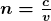, where  is the speed of light in the material, c is the speed of light in vacuum, and n is the index of refraction.
• Snell’s law, the law of refraction, is stated in equation form as n1 sin θ1 = n2 sin θ2.

### Conceptual Questions

1: Diffusion by reflection from a rough surface is described in this chapter. Light can also be diffused by refraction. Describe how this occurs in a specific situation, such as light interacting with crushed ice.

2: Why is the index of refraction always greater than or equal to 1?

3: Does the fact that the light flash from lightning reaches you before its sound prove that the speed of light is extremely large or simply that it is greater than the speed of sound? Discuss how you could use this effect to get an estimate of the speed of light.

4: Will light change direction toward or away from the perpendicular when it goes from air to water? Water to glass? Glass to air?

5: Explain why an object in water always appears to be at a depth shallower than it actually is? Why do people sometimes sustain neck and spinal injuries when diving into unfamiliar ponds or waters?

6: Explain why a person’s legs appear very short when wading in a pool. Justify your explanation with a ray diagram showing the path of rays from the feet to the eye of an observer who is out of the water.

7: Why is the front surface of a thermometer curved as shown?

8: Suppose light were incident from air onto a material that had a negative index of refraction, say –1.3; where does the refracted light ray go?

### Problems & Exercises

1: What is the speed of light in water? In glycerine?

2: What is the speed of light in air? In crown glass?

3: Calculate the index of refraction for a medium in which the speed of light is 2.012 x 10 m/s, and identify the most likely substance based on Table 1.

4: In what substance in Table 1 is the speed of light 2.290 x 108 m/s?

5: There was a major collision of an asteroid with the Moon in medieval times. It was described by monks at Canterbury Cathedral in England as a red glow on and around the Moon. How long after the asteroid hit the Moon, which is 3.84 x 108 m away, would the light first arrive on Earth?

6: A scuba diver training in a pool looks at his instructor as shown in Figure 5. What angle does the ray from the instructor’s face make with the perpendicular to the water at the point where the ray enters? The angle between the ray in the water and the perpendicular to the water is 25.0 degrees.

7: Components of some computers communicate with each other through optical fibres having an index of refraction n = 1.55. What time in nanoseconds is required for a signal to travel 0.200 m through such a fibre?

8: (a) Using information in Figure 5, find the height of the instructor’s head above the water, noting that you will first have to calculate the angle of incidence. (b) Find the apparent depth of the diver’s head below water as seen by the instructor.

9: Suppose you have an unknown clear substance immersed in water, and you wish to identify it by finding its index of refraction. You arrange to have a beam of light enter it at an angle of 45.0 degrees, and you observe the angle of refraction to be 40.3 degrees. What is the index of refraction of the substance and its likely identity?

10: On the Moon’s surface, lunar astronauts placed a corner reflector, off which a laser beam is periodically reflected. The distance to the Moon is calculated from the round-trip time. What percent correction is needed to account for the delay in time due to the slowing of light in Earth’s atmosphere? Assume the distance to the Moon is precisely 3.84 x108 m , and Earth’s atmosphere (which varies in density with altitude) is equivalent to a layer 30.0 km thick with a constant index of refraction n=1.000293.

11: Suppose Figure 6 represents a ray of light going from air through crown glass into water, such as going into a fish tank. Calculate the amount the ray is displaced by the glass (Δx), given that the incident angle is 40.0 o and the glass is 1.00 cm thick.

12: Figure 6 shows a ray of light passing from one medium into a second and then a third. Show that θ3 is the same as it would be if the second medium were not present (provided total internal reflection does not occur).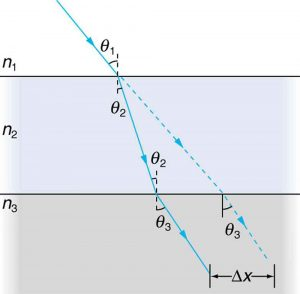Figure 6. A ray of light passes from one medium to a third by traveling through a second. The final direction is the same as if the second medium were not present, but the ray is displaced by Δx (shown exaggerated).

13: Unreasonable Results

Suppose light travels from water to another substance, with an angle of incidence of 10.0and an angle of refraction of 14.9o (a) What is the index of refraction of the other substance? (b) What is unreasonable about this result? (c) Which assumptions are unreasonable or inconsistent?

Consider sunlight entering the Earth’s atmosphere at sunrise and sunset—that is, at a 90.0o incident angle. Taking the boundary between nearly empty space and the atmosphere to be sudden, calculate the angle of refraction for sunlight. This lengthens the time the Sun appears to be above the horizon, both at sunrise and sunset. Now construct a problem in which you determine the angle of refraction for different models of the atmosphere, such as various layers of varying density. Your instructor may wish to guide you on the level of complexity to consider and on how the index of refraction varies with air density.

15: Unreasonable Results

Light traveling from water to a gemstone strikes the surface at an angle of 80.0o and has an angle of refraction of 15.2o. (a) What is the speed of light in the gemstone? (b) What is unreasonable about this result? (c) Which assumptions are unreasonable or inconsistent?

## Glossary

refraction
changing of a light ray’s direction when it passes through variations in matter
index of refraction
for a material, the ratio of the speed of light in vacuum to that in the material

### Solutions

Problems & Exercises

1: 2.25 x 10 m/s  in water, 2.04 x 108 m/s in glycerine

2: v air = 2.9964×108 m/s,  v crown glass = 1.97 x108 m/s

3: 1.49, polystyrene

4: 1.31, ice at 0 oC

5: 1.28 s

6: 34.2o

7: 1.03 ns

8: a) 34.29o , 2.93 m b) 1.37 m

9: n=1.46 fused quartz

10: 2.29 x 10-6 %

11: 25.02o  3.72 x 10-3 m

13: (a) 0.898 (b) Can’t have n < 1.00 since this would imply a speed greater than c. (c) Refracted angle is too big relative to the angle of incidence.

15: (a) c/ 5.00 (b) Speed of light too slow, since index is much greater than that of diamond. (c) Angle of refraction is unreasonable relative to the angle of incidence.